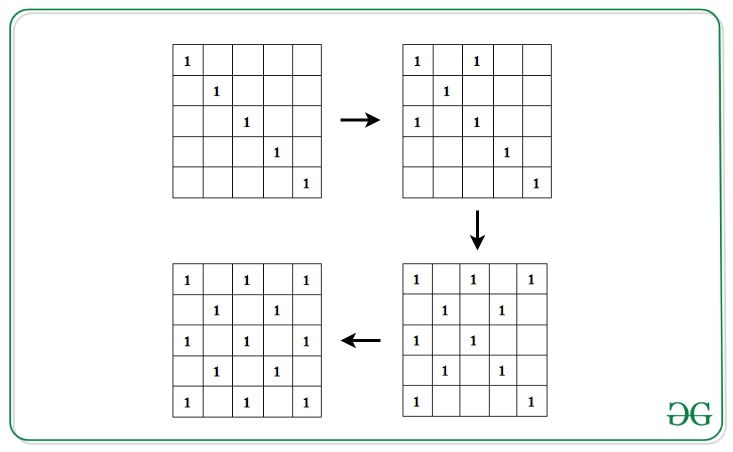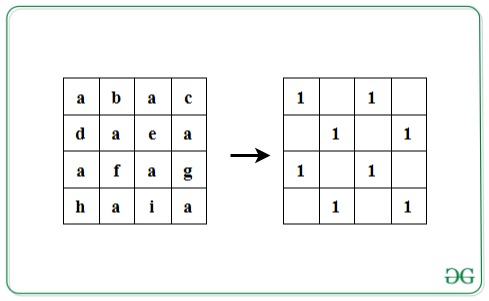Check if given string satisfies the following conditions

• Last Updated : 02 Dec, 2021

Given a string S of length L, where L is a perfect square, the task is to check if the given string satisfies the following conditions:

• Insert the characters of the string into a square matrix A[][] of dimensions √L x √L in row-wise manner.
• Initialize another matrix M[][] with 0s. Fill the left diagonal with 1. Now, for 1 present in the left diagonal, fill its corresponding right diagonal with 1s.• Now, check if all the indices in matrix M[][] which contains 1, contains the same character in A[][].

If the condition is satisfied, print “Yes”. Otherwise, print “No”.

Examples:

Input: S = ”abacdaeaafaghaia”
Output: Yes
Explanation:Input: S = ”abacdaeabfaghaia”
Output: No

Approach: The idea is to traverse the matrix A[][] where its corresponding character in the matrix M[][] is 1. Follow the steps below to solve the problem:

• Calculate the dimensions of the matrix as N = √L.
• Iterate over the left diagonal by visiting each cell, A[i][i] where 1<= i<= N.
• For every element of the left diagonal at cell A[i][i], initialize variables x and y with i and traverse its corresponding right diagonal by visiting the character S[x*N + y] and S[y*N + x] and decrement x each time by 1 and increment y each time by 1 to move along the next cells in the right diagonals, while x is not smaller than 0 and y is smaller than N.
• If all characters are found to be same in the above step, print “Yes”. Otherwise, print “No” if any mismatch is found.

Below is the implementation of the above approach:

C++

 // C++ program for the above approach #include using namespace std; // Function to check if given string// satisfies the given conditionsvoid isValid(string s){     // Dimensions    int n = sqrt(s.length());     char check = s;     // Left diagonal    for (int i = 0; i < n; i++) {        int x = i, y = i;         // Right diagonal        while (x >= 0 && y < n) {            if (s[(n * x) + y] != check                || s[(n * y) + x] != check) {                 // Conditions not satisfied                cout << "No" << endl;                return;            }            x--;            y++;        }    }     // Print Yes    cout << "Yes" << endl;} // Driver Codeint main(){     // Given String    string str = "abacdaeaafaghaia";     // Function call    isValid(str);     return 0;}

Java

 // Java program for the above approachimport java.util.*;   class GFG{   // Function to check if given string// satisfies the given conditionsstatic void isValid(String s){         // Dimensions    int n = (int)Math.sqrt(s.length());          char check = s.charAt(0);          // Left diagonal    for(int i = 0; i < n; i++)    {        int x = i, y = i;                 // Right diagonal        while (x >= 0 && y < n)        {            if (s.charAt((n * x) + y) != check ||                s.charAt((n * y) + x) != check)            {                                 // Conditions not satisfied                System.out.print("No");                return;            }            x--;            y++;        }    }         // Print Yes    System.out.print("Yes");}   // Driver Codepublic static void main(String[] args){         // Given String    String str = "abacdaeaafaghaia";         // Function call    isValid(str);}} // This code is contributed by code_hunt

Python3

 # Python3 program for the above approachimport math # Function to check if given string# satisfies the given conditionsdef isValid(s):         # Dimensions    n = int(math.sqrt(len(s)))    check = s     # Left diagonal    for i in range(n):        x = i        y = i                 # Right diagonal        while (x >= 0 and y < n):            if (s[n * x + y] != check or                s[n * x + x] != check):                                     # Conditions not satisfied                print("No")                return                             x -= 1            y += 1                 # Print Yes    print("Yes")     # Driver Code # Given Stringstr = "abacdaeaafaghaia" # Function callisValid(str) # This code is contributed by avanitrachhadiya2155

C#

 // C# program for the above approach using System;  class GFG{  // Function to check if given string// satisfies the given conditionsstatic void isValid(string s){         // Dimensions    int n = (int)Math.Sqrt(s.Length);         char check = s;         // Left diagonal    for(int i = 0; i < n; i++)    {        int x = i, y = i;                 // Right diagonal        while (x >= 0 && y < n)        {            if (s[(n * x) + y] != check ||                s[(n * y) + x] != check)            {                                 // Conditions not satisfied                Console.Write("No");                return;            }            x--;            y++;        }    }      // Print Yes    Console.Write("Yes");} // Driver codepublic static void Main(){         // Given String    string str = "abacdaeaafaghaia";      // Function call    isValid(str);}} // This code is contributed by sanjoy_62

Javascript


Output:
Yes

Time Complexity: O(L) where L is the length of the given string.
Auxiliary Space: O(L)

My Personal Notes arrow_drop_up